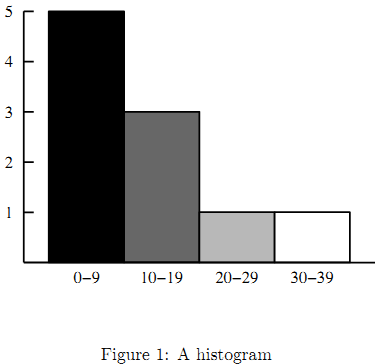Online JudgeProblem SetAuthorsOnline ContestsUser
Web Board
F.A.Qs
Statistical Charts
Problems
Submit Problem
Online Status
Prob.ID:
Register
Authors ranklist
Current Contest
Past Contests
Scheduled Contests
Award Contest
Register
Language:
Grey Area
 Time Limit: 1000MS Memory Limit: 65536K Total Submissions: 310 Accepted: 153 Special Judge

Description

Dr. Grey is a data analyst, who visualizes various aspects of data received from all over the world everyday. He is extremely good at sophisticated visualization tools, but yet his favorite is a simple self-made histogram generator.
Figure 1 is an example of histogram automatically produced by his histogram generator. A histogram is a visual display of frequencies of value occurrences as bars. In this example, values in the interval 0–9 occur five times, those in the interval 10–19 occur three times, and 20–29 and 30–39 once each.Dr. Grey’s histogram generator is a simple tool. First, the height of the histogram is fixed, that is, the height of the highest bar is always the same and those of the others are automatically adjusted proportionately. Second, the widths of bars are also fixed. It can only produce a histogram of uniform intervals, that is, each interval of a histogram should have the same width (10 in the above example). Finally, the bar for each interval is painted in a grey color, where the colors of the leftmost and the rightmost intervals are black and white, respectively, and the darkness of bars monotonically decreases at the same rate from left to right. For instance, in Figure 1, the darkness levels of the four bars are 1, 2=3, 1=3, and 0, respectively.
In this problem, you are requested to estimate ink consumption when printing a histogram on paper. The amount of ink necessary to draw a bar is proportional to both its area and darkness.

Input

The input consists of multiple datasets, each of which contains integers and specifies a value table and intervals for the histogram generator, in the following format.

n w
v1
v2
.
.
.
vn

n is the total number of value occurrences for the histogram, and each of the n lines following the first line contains a single value. Note that the same value may possibly occur multiple times.
w is the interval width. A value v is in the first (i.e. leftmost) interval if 0 ≤ v < w, the second one if w ≤ v < 2w, and so on. Note that the interval from 0 (inclusive) to w (exclusive) should be regarded as the leftmost even if no values occur in this interval. The last (i.e. rightmost) interval is the one that includes the largest value in the dataset.
You may assume the following.

1 ≤ n ≤ 100
10 ≤ w ≤ 50
0 ≤ vi ≤ 100 for 1 ≤ i ≤ n

You can also assume that the maximum value is no less than w. This means that the histogram has more than one interval.
The end of the input is indicated by a line containing two zeros.

Output

For each dataset, output a line containing the amount of ink consumed in printing the histogram.
One unit of ink is necessary to paint one highest bar black. Assume that 0:01 units of ink per
histogram is consumed for various purposes except for painting bars such as drawing lines and
characters (see Figure 1). For instance, the amount of ink consumed in printing the histogram
in Figure 1 is:Each output value should be in a decimal fraction and may have an error less than 10-5.

Sample Input

3 50
100
0
100
3 50
100
100
50
10 10
1
2
3
4
5
16
17
18
29
30
0 0

Sample Output

0.51
0.26
1.4766666666666667

Source

[Submit]   [Go Back]   [Status]   [Discuss]Home PageGo BackTo top